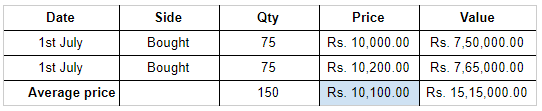Upstox uses the First In, First Out (FIFO) method for calculating the average price for overnight (Delivery) positions. Let’s understand this calculation by taking an example of Nifty Futures.

On 1st July:Orders placed: 2

1st order: Quantity = 225 | Price = Rs. 10,000.00

2nd order: Quantity = 75 | Price = Rs. 10,200.00

To calculate the average price, first calculate the value (Quantity x Price). Hence:

1st trade: Rs. 22,50,000.00

2nd trade: Rs. 7,65,000.00

Total quantity = 300

Total value: Rs. 30,15,000.00

Divide total value by total quantity:

Rs. 30,15,000.00 ÷ 300 = Rs.10,050.00

This is the average price.

On 5th July:Let us see what happens when you add a sell order to this:

Sell order placed on 5th July: 150 (out of 300)

Price: Rs. 9,950.00

Now the FIFO method will be applied here. The method will check the first trade (on the buy side). In this case, it is 225. 150 will be deducted from 225. The balance left is shown below.

After applying the FIFO method,

Balance: 225 - 150 = 75(Note: In case the sell quantity was more than 225, then it would have moved to the next trade to deduct the remaining quantity)

Average price = Total Price ÷ Total Quantity

Average price: Rs. 15,15,000.00 ÷ 150 = Rs. 10,100.00

This is how the FIFO method is used for calculating the average price. Calculation remains the same even if you are carrying it on the short sold position instead of the buy position.

If your query is still not resolved, please raise a ticket here.